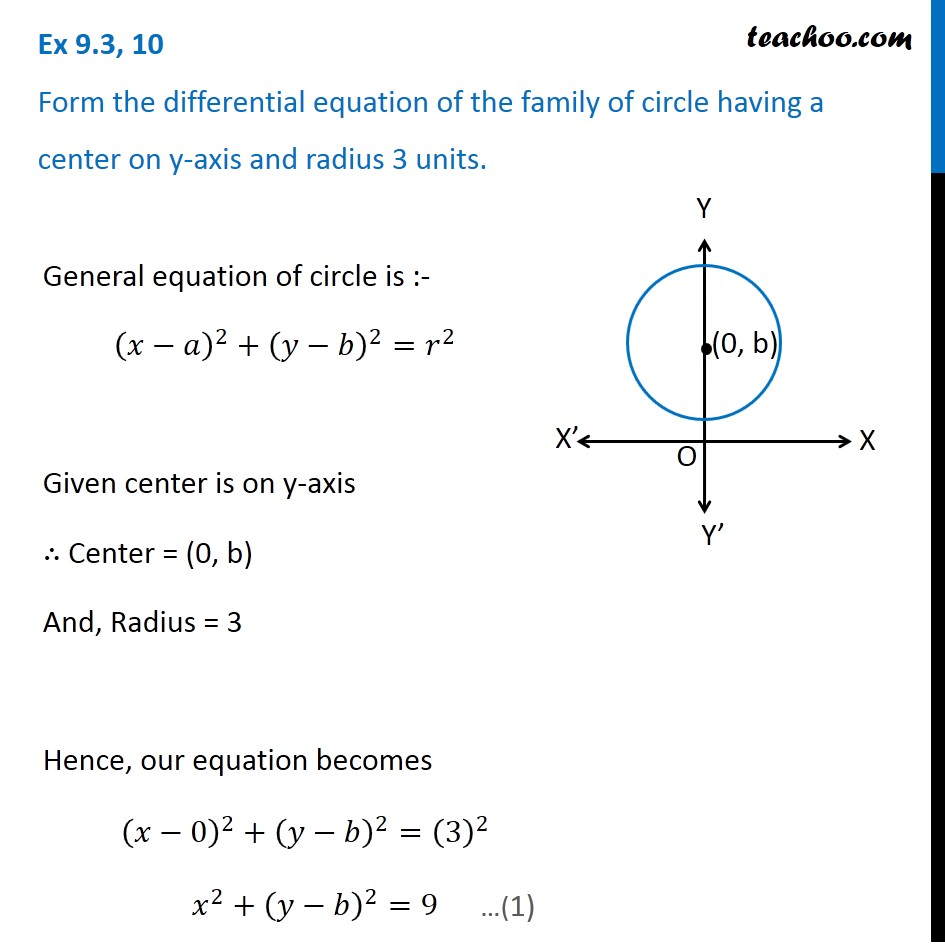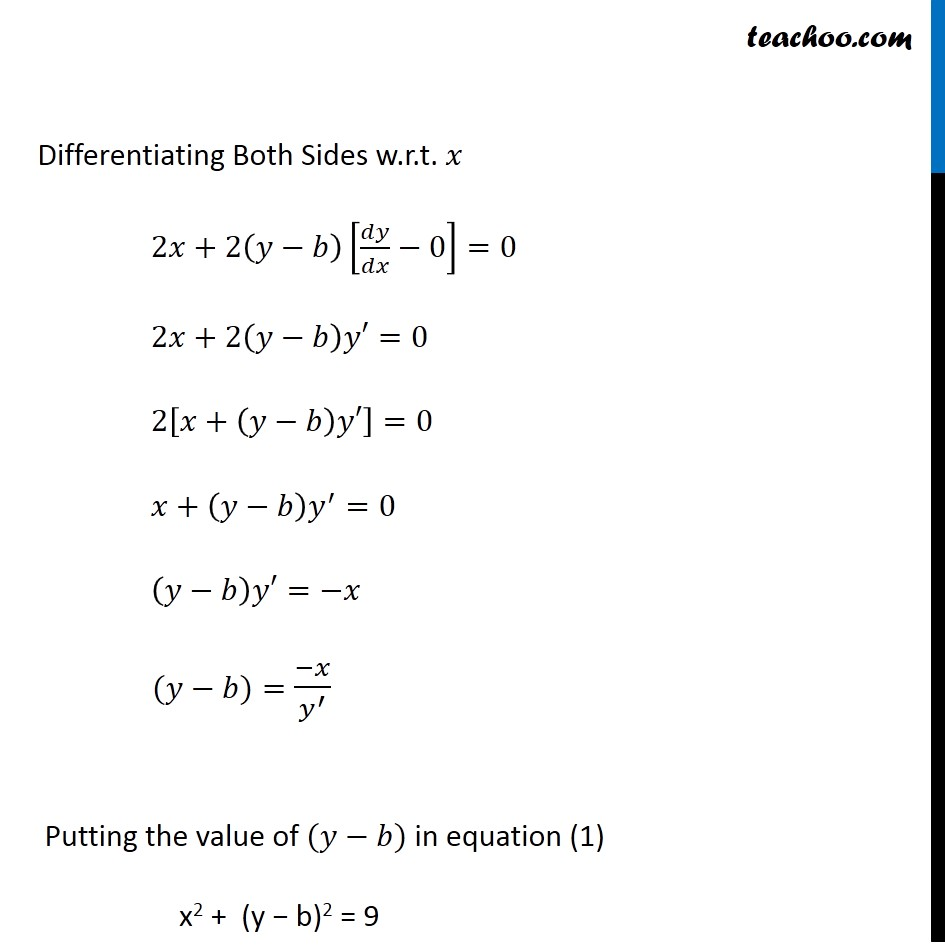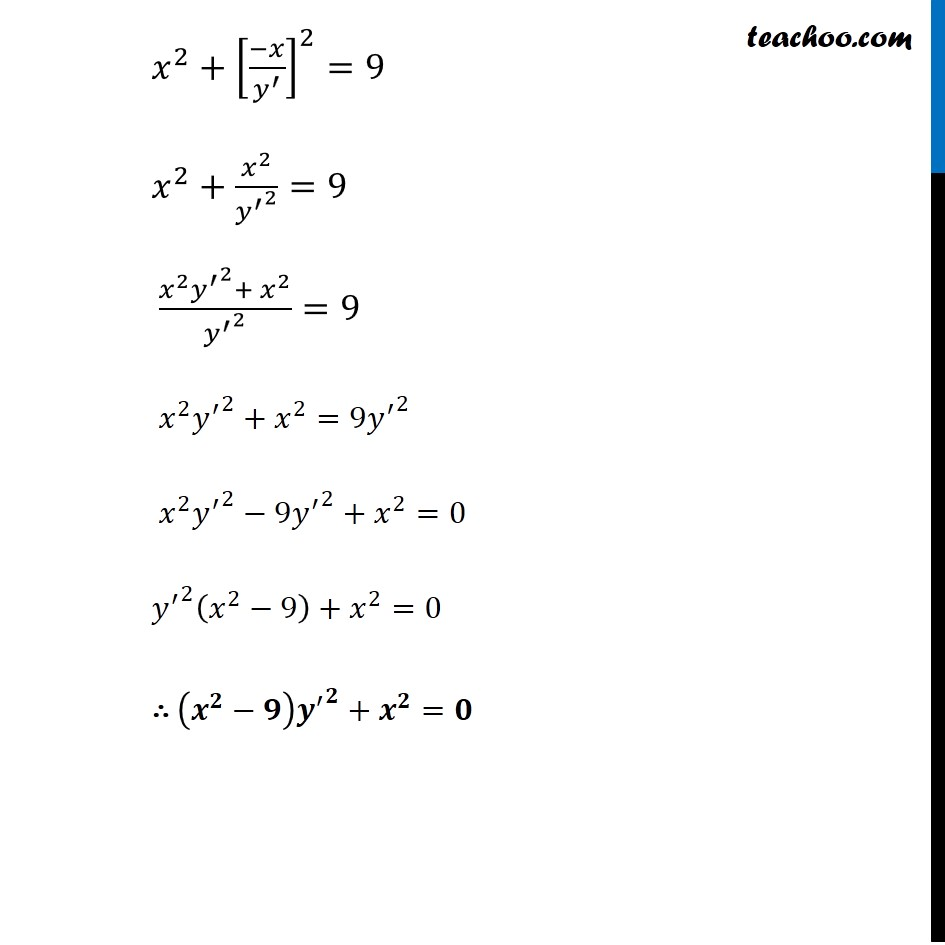Forming Differential equations

Chapter 9 Class 12 Differential Equations
Serial order wiseLearn in your speed, with individual attention - Teachoo Maths 1-on-1 Class

### Transcript

Question 10 Form the differential equation of the family of circle having a center on y-axis and radius 3 units. General equation of circle is :- (𝑥−𝑎)^2+(𝑦−𝑏)^2=𝑟^2 Given center is on y-axis ∴ Center = (0, b) And, Radius = 3 Hence, our equation becomes (𝑥−0)^2+(𝑦−𝑏)^2=(3)^2 𝑥^2+(𝑦−𝑏)^2=9 Differentiating Both Sides w.r.t. 𝑥 2𝑥+2(𝑦−𝑏)[𝑑𝑦/𝑑𝑥−0]=0 2𝑥+2(𝑦−𝑏)𝑦′=0 2[𝑥+(𝑦−𝑏)𝑦′]=0 𝑥+(𝑦−𝑏) 𝑦^′=0 (𝑦−𝑏)𝑦′=−𝑥 (𝑦−𝑏)= (−𝑥)/𝑦^′ Putting the value of (𝑦−𝑏) in equation (1) x2 + (y − b)2 = 9 𝑥^2+[(−𝑥)/𝑦^′ ]^2=9 𝑥^2+𝑥^2/〖𝑦^′〗^2 =9 (𝑥^2 〖𝑦^′〗^2+ 𝑥^2)/〖𝑦^′〗^2 =9 𝑥^2 〖𝑦^′〗^2+𝑥^2=9〖𝑦^′〗^2 𝑥^2 〖𝑦^′〗^2−9〖𝑦^′〗^2+𝑥^2=0 〖𝑦^′〗^2 (𝑥^2−9)+𝑥^2=0 ∴ (𝒙^𝟐−𝟗) 〖𝒚^′〗^𝟐+𝒙^𝟐=𝟎